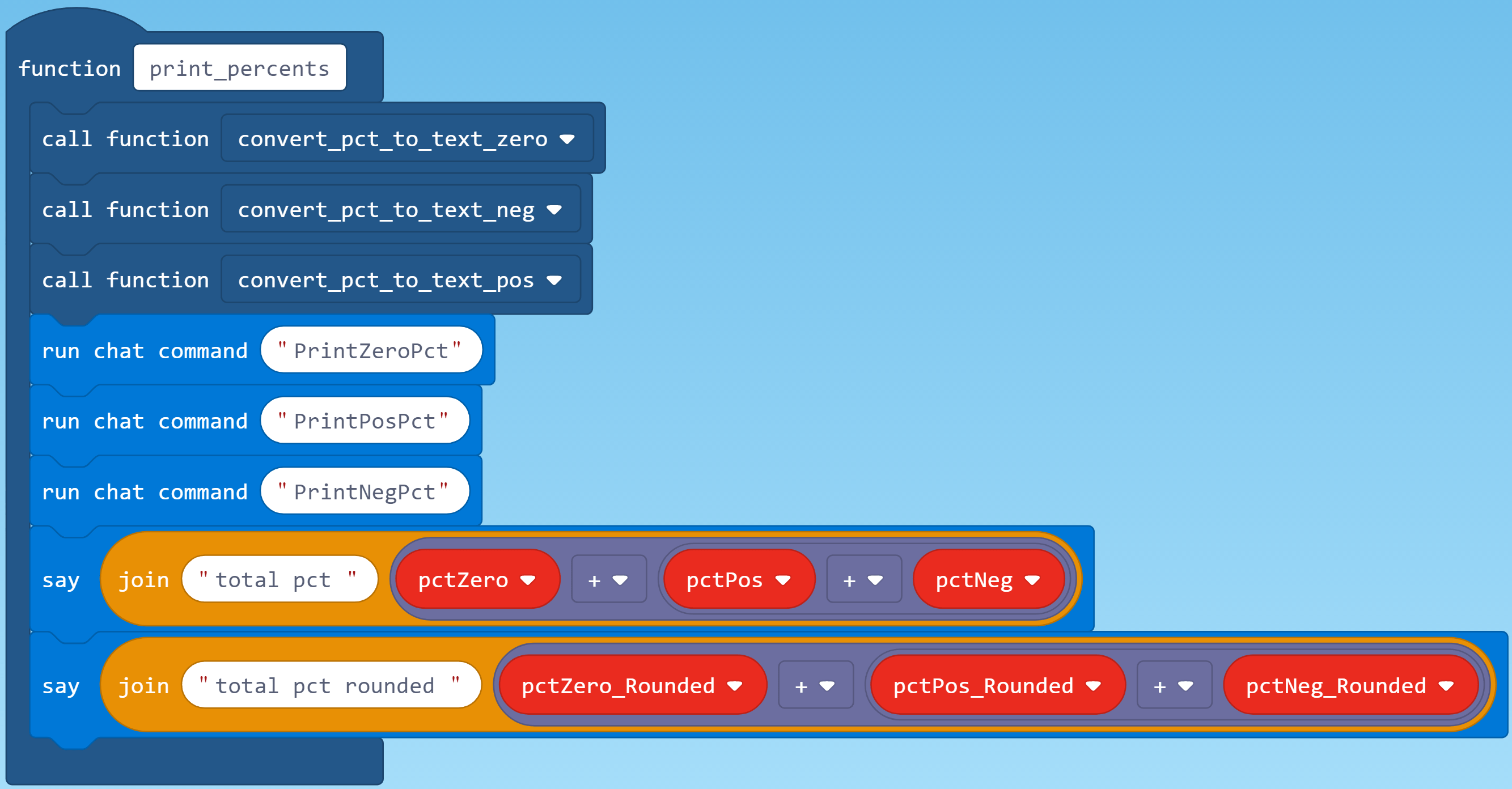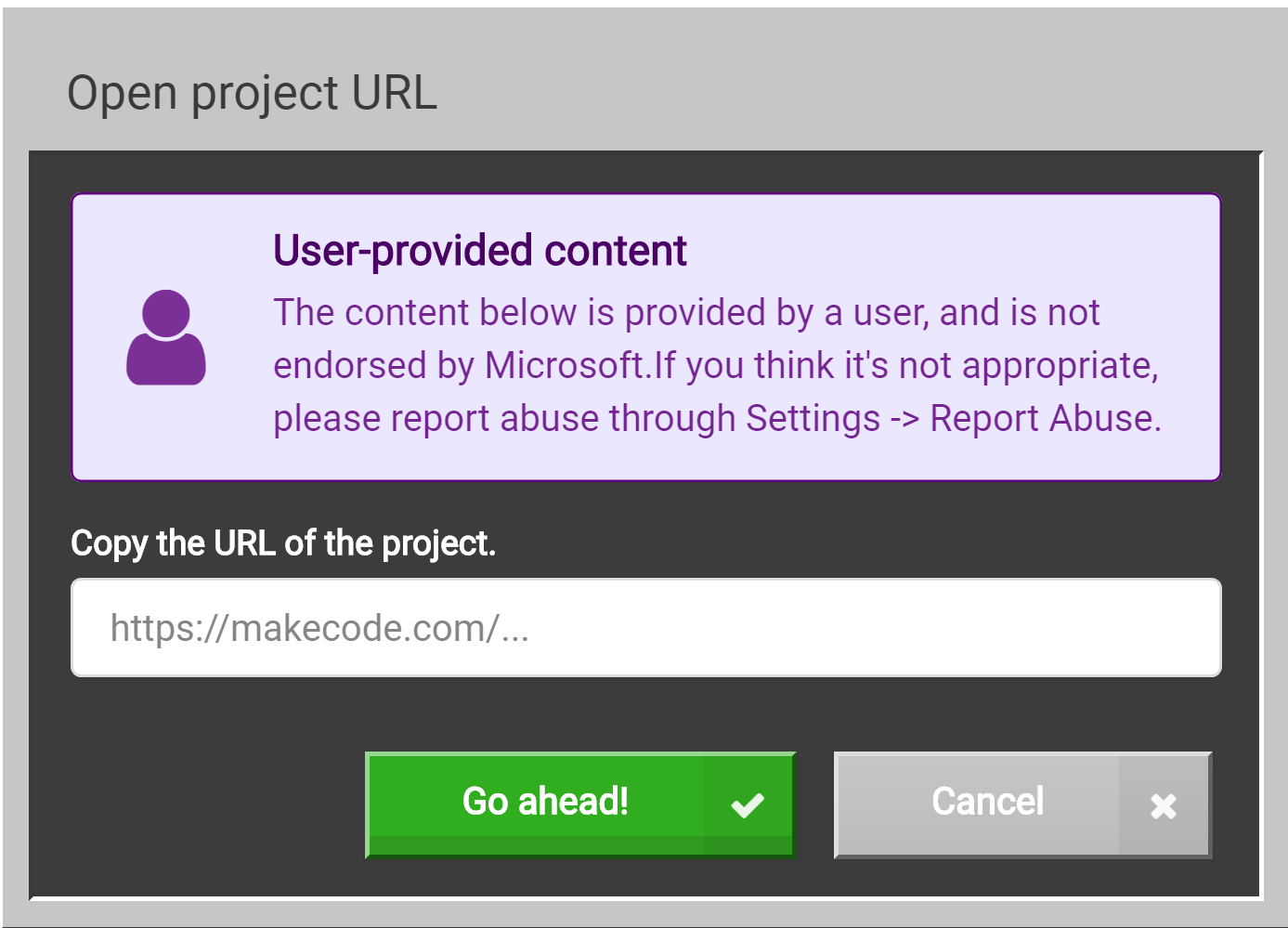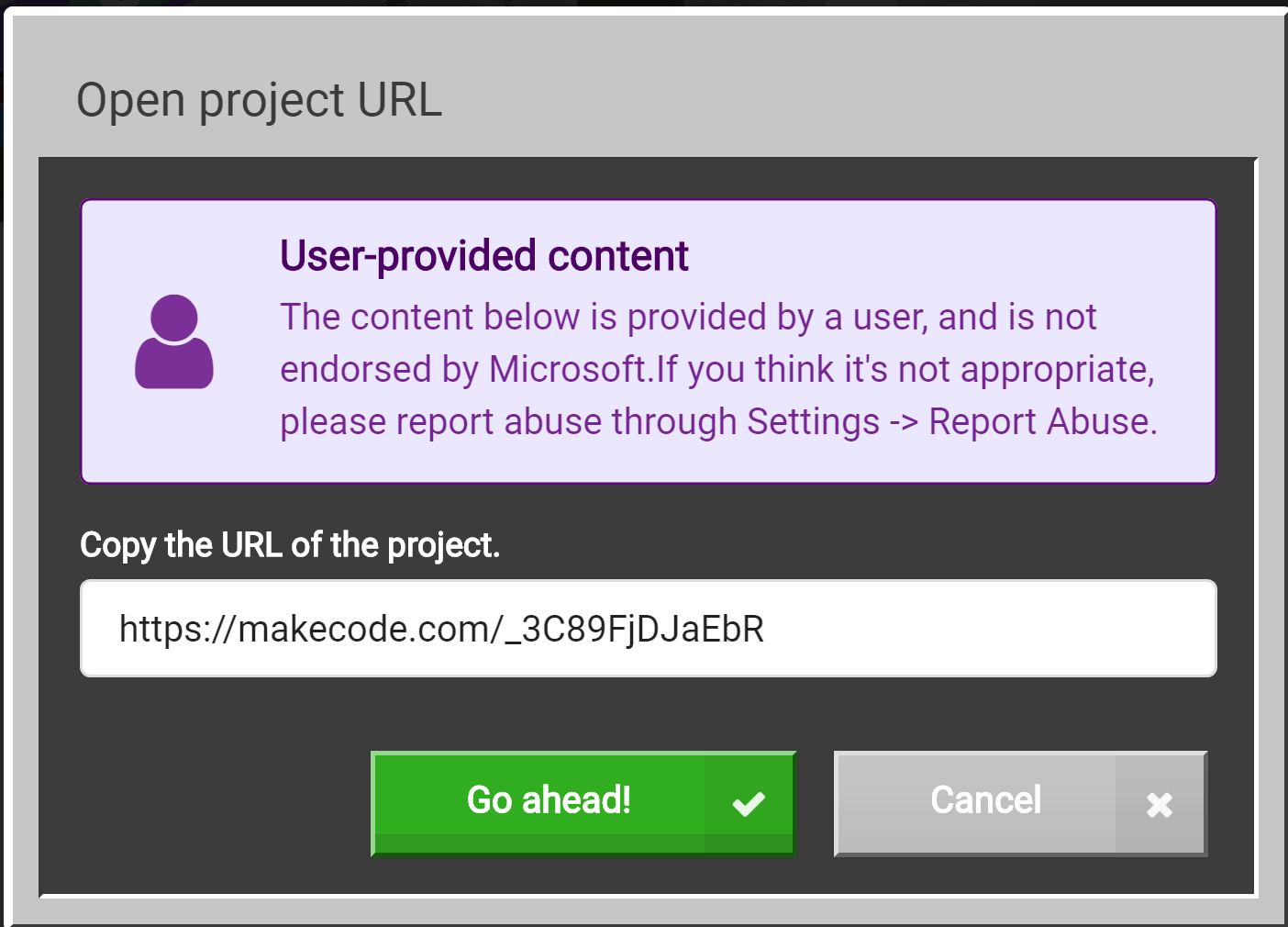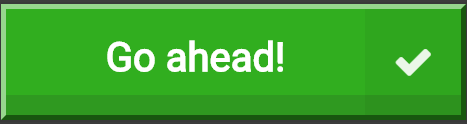# Negative Random Number Tester in MakeCode for MinecraftI have made several uses of negative random numbers in my MakeCode for Minecraft programs, for example, in Coordinates Tutor in MakeCode for Minecraft I used random numbers between -5 and +5 to generates X and Z coordinates on a grid. With each use,  I wondered whether the number of negative numbers generated were, in fact, equal to the number of positive numbers generated. Thus, this exploration.

By experimenting with this program, I observed two things. First, in cases where I generated fewer random numbers, say 10, the number of positive numbers and negative numbers were often quite different. For example, in one case of only 10 generated numbers, 60% were positive and 30% were negative — not close. However, in the case of a million random numbers, 47% were positive  and 48% were negative — close. But, remember that in both cases, because the numbers are random, different runs of the program may give different results.

Three runs of the program generating 10 random numbers each gave the results shown below. The case on the left was perfect with exactly the same negative as positive numbers. The other two cases were not so close. Generating only 10 numbers is likely to give results like on the right because the number of runs (the sample size  n) is small and, thus,  one can expect large variation in the cases.

Three runs of the program with But, remember that in both cases, because the numbers are random, different runs of the program may give different results. random numbers gave the results shown below. The case on the left yielded exactly the same negative as positive numbers. The other two cases were not exact; but, they were close. Because the sample size n 100,000  is relative large, the variation in the runs is not great. But, beware, a single run might have very different results.

While the two cases on the right (above) total to 100%, the case on the left totals to 101%. This is caused by

1. division that may not yield a whole number and
2. by rounding.

This is illustrated below, which is say-block output from the program. These results show more significant digits than the billboard output.. Notice on the right that while before rounding the total is 100% but after rounding the calculated percentages is 101%.

Don’t worry if the reasons for such situations are not intuitive. Entire books are written on the subject. I remember taking and later teaching courses called “Machine Arithmetic” and  “Numerical Analysis,” which tediously explored such situation.  In your programming journey, just know that such situations exists and devise code to give the precision needed.

## The Plan

The plan for Negative Random Number Tester, or just “Tester,” is to generate some number n random numbers between negative and positive limits of equal absolute value; for example from -10 to +10 or from -1000 to +1000 or from -1 to +1. The exact value of the limits should not matter; however, I did not test that.

The number of random numbers generated n is an argument to the run on chat command that starts the program. It can have values from 10 to 1,000,000.

The guts of the program is the loop that generates the random numbers counting individually the number of zero, positive and negative numbers.

After all the numbers have been generated and counted, the n and the percentages of each of zero, positive and negative random numbers are printed on a billboard, as shown above.

## The Code

The run on-chat command is on the top left. The right half of the screen are those routines that convert counts to percentages and print them on the billboard.The run on chat command performs the following:

1. Calls a function to move the player, set the origin and set the print position on the billboard.
2. Checks the argument n for the correct range of 10 to 1,000,000. If n is out of range it set it to the default value 100,000.
3. Builds the billboard on which the results will be printed.
4. Initializes the count variables for zero, positive and negatives values of generated random numbers.
5. Loops n times generating random numbers and counting the resulting values that are zero, positive or negative.
6. After exiting the loop, says the three count values and calls a function to convert and print them on the billboard.Rather than print results in the sky, they are printing on a billboard..The routine in charge of printing the percentages first calls the functions that convert each of the percentages to text. It then runs the three chat commands to do the actual printing. It also says two totals, one for the total of the three percentages before rounding and one for after rounding.There are three individual function for the conversions for no other reasons than to organize the code. The routines doe the following:

1. calculates count as percentage of total,
2. rounds the result,
3. calls the format_pct routine right align the number in a 3-digit string, and
4. adds the appropriate label and the % sign.

The gray block indicates that rounding is done in JavaScript.The format_pct routine takes as input a text variable representation of an integer percentage; for example, “1” or “13” or “100”. Since an integer percentage may have 1, 2 or 3 digits, it makes the forces the string to be length  3 by adding spaces on the left of the digits. When the numbers so modified are printed in a column, as they are on the billboard, they will be right aligned.The print routines are individual chat commands, not functions, so that they will print simultaneously, which greatly speeds up the print. Notice that when the program is running the three numbers print at the same time. Each of the print routines have a different Y-axis value, which specifies the up-down position on the billboard..This code assumes a flat world such as that described in MakeCode for Minecraft Sandbox World: Make It Flat and Simple.

## Get the Code

Negative Random Number Tester code is shared on Code Connection at this URL

https://makecode.com/_EfC4c9WCUcuR

To get and use the code, follow these steps.

Click the Import button, which is upper right in the Code Connection window just below the banner. This will open the window shown below.Click the Import URL button, which is on the right, to open the window shown below.Paste the URL supplied for the program you want to use in the space under the text “Copy the URL …”Click the Go ahead! button.

The next window you will see will be the MakeCode window with the code downloaded from the URL. At this point, you can treat it like any other code, e.g., run it, save it locally, modify it, publish your changes or whatever else your heat desires.

We have tested several other methods of downloading the code using the URL, for example, pasting the URL in a browser. No joy. For more detailed instruction see our post How to Use Shared MakeCode on Microsoft Code Connection for Minecraft.